JEE  >  Competition Level Test: Application of Derivative- 2

# Competition Level Test: Application of Derivative- 2

Test Description

## 30 Questions MCQ Test Mathematics For JEE | Competition Level Test: Application of Derivative- 2

Competition Level Test: Application of Derivative- 2 for JEE 2023 is part of Mathematics For JEE preparation. The Competition Level Test: Application of Derivative- 2 questions and answers have been prepared according to the JEE exam syllabus.The Competition Level Test: Application of Derivative- 2 MCQs are made for JEE 2023 Exam. Find important definitions, questions, notes, meanings, examples, exercises, MCQs and online tests for Competition Level Test: Application of Derivative- 2 below.
Solutions of Competition Level Test: Application of Derivative- 2 questions in English are available as part of our Mathematics For JEE for JEE & Competition Level Test: Application of Derivative- 2 solutions in Hindi for Mathematics For JEE course. Download more important topics, notes, lectures and mock test series for JEE Exam by signing up for free. Attempt Competition Level Test: Application of Derivative- 2 | 30 questions in 60 minutes | Mock test for JEE preparation | Free important questions MCQ to study Mathematics For JEE for JEE Exam | Download free PDF with solutions
 1 Crore+ students have signed up on EduRev. Have you?
Competition Level Test: Application of Derivative- 2 - Question 1

### The value of ‘a’ for which x3 - 3x + a = 0 has two distinct roots in [0, 1] is given by

Detailed Solution for Competition Level Test: Application of Derivative- 2 - Question 1

Let α, β ∈[0,1].f (x) is continuous on [a,b] & differentiable on (a,b) and f (α) = f (β) = 0
∴ c ∈ (α, β) such that f' (c) = 0 ⇒ c = ±1∉ (0,1)

Competition Level Test: Application of Derivative- 2 - Question 2

### The value of ‘c’ in Lagrange’s mean value theorem for f (x) = x (x- 2)2 in [0, 1]

Detailed Solution for Competition Level Test: Application of Derivative- 2 - Question 2

f '(c) = 0 2c(c - 2) + (c - 2)2 = 0
c = 2,2/3
∴ c = 2/3 (c ≠2)

Competition Level Test: Application of Derivative- 2 - Question 3

### For the function f (x) = x3 - 6x2 + ax + b, if Roll’s theorem holds in [1, 3] with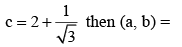Detailed Solution for Competition Level Test: Application of Derivative- 2 - Question 3

f (1) = f (3) ⇒ a = 11

Competition Level Test: Application of Derivative- 2 - Question 4

Find Value of ‘c’ by using  Rolle’s theorem for f (x) = log (x2 + 2) - log 3 on [-1,1]

Detailed Solution for Competition Level Test: Application of Derivative- 2 - Question 4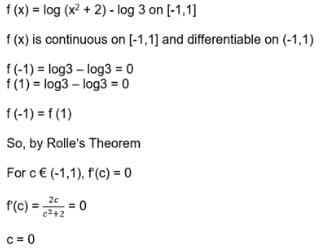Competition Level Test: Application of Derivative- 2 - Question 5

The chord joining the points where x = p and x = q on the curve y = ax2 + bx + c is parallel to the tangent at the point on the curve whose abscissa is

Detailed Solution for Competition Level Test: Application of Derivative- 2 - Question 5

Apply Lagrange’s theorm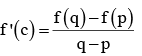Competition Level Test: Application of Derivative- 2 - Question 6

The least value of k for which the function f(x) = x2 + kx + 1 is a increasing  function in the interval 1 < x < 2

Detailed Solution for Competition Level Test: Application of Derivative- 2 - Question 6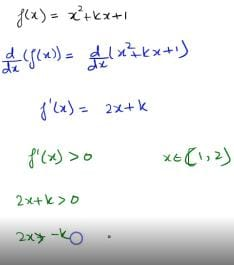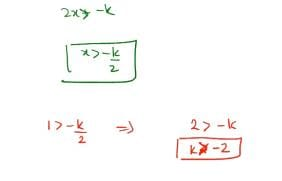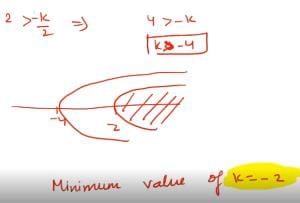Competition Level Test: Application of Derivative- 2 - Question 7

The interval in  which f (x) = x3 - 3x2 - 9x + 20 is strictly decreasing

Detailed Solution for Competition Level Test: Application of Derivative- 2 - Question 7

Given f (x) = x3 - 3x2 - 9x + 20
⇒ f '(x) = 3x2 -6x -9
⇒ f '(x) = 3(x - 3)(x +1)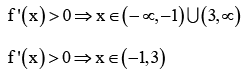Thus, f (x) is strictly increasing for
x ∈ (-∞,-1) U (3, ∞) and strictly decreasing for x ∈ (-1, 3)

Competition Level Test: Application of Derivative- 2 - Question 8

The critical points of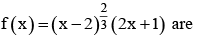Detailed Solution for Competition Level Test: Application of Derivative- 2 - Question 8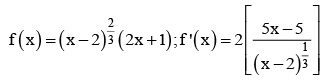f ' (x) = 0 ⇒ x = 1; f1 (x) does not exist at x = 2
∴ x = 1 and x = 2 are two critical points

Competition Level Test: Application of Derivative- 2 - Question 9

The number of stationary points of f (x) = sin x in [0,2π] are

Detailed Solution for Competition Level Test: Application of Derivative- 2 - Question 9

f (x) = sinx ⇒ f '(x) = cosx ⇒ f '(x) = 0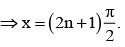Therefore number of stationary points of f (x) in [0, 2π] is 2.

Competition Level Test: Application of Derivative- 2 - Question 10

Local minimum values of the function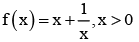Detailed Solution for Competition Level Test: Application of Derivative- 2 - Question 10

AM > GM

Competition Level Test: Application of Derivative- 2 - Question 11

If the function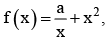has maximum at x =-3, then the value of ‘a’ is

Detailed Solution for Competition Level Test: Application of Derivative- 2 - Question 11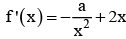since f (x) has local maximum at x = -3 ⇒ f ' (-3) = 0 and f 11 (-3)< 0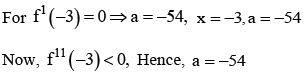Competition Level Test: Application of Derivative- 2 - Question 12

The point at which f (x) = (x- 1)4 assumes local maximum or local minimum value are

Detailed Solution for Competition Level Test: Application of Derivative- 2 - Question 12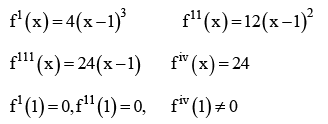Therefore n = iv is even and fiv (1) = 24> 0
Therefore f (x) has local minimum at x = 1.

Competition Level Test: Application of Derivative- 2 - Question 13

The global maximum and global minimum of f (x) = 2x3 - 9x2 + 12x + 6 in [0, 2]

Detailed Solution for Competition Level Test: Application of Derivative- 2 - Question 13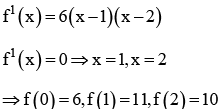Therefore global maximum M1 = max{f (0), f (1), f (2)}= 11
Global minimum
M2 = max{f (0), f (1), f (2)}= 6

Competition Level Test: Application of Derivative- 2 - Question 14

The approximate value of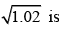Detailed Solution for Competition Level Test: Application of Derivative- 2 - Question 14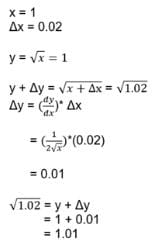Competition Level Test: Application of Derivative- 2 - Question 15

The approximate value of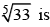Detailed Solution for Competition Level Test: Application of Derivative- 2 - Question 15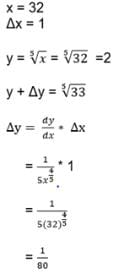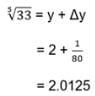Competition Level Test: Application of Derivative- 2 - Question 16

If the percentage error in the surface area of sphere is k, then the percentage error in its volume is

Detailed Solution for Competition Level Test: Application of Derivative- 2 - Question 16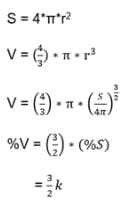Competition Level Test: Application of Derivative- 2 - Question 17

If an error of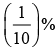is made in measuring the radius of a sphere then percentage error in its volume is

Detailed Solution for Competition Level Test: Application of Derivative- 2 - Question 17

V% = 3(S%)

Competition Level Test: Application of Derivative- 2 - Question 18

The height of a cylinder is equal to its radius. If an error of 1 % is made in its height. Then the percentage error in its volume is

Detailed Solution for Competition Level Test: Application of Derivative- 2 - Question 18

h = r and v = ph3; V% = 3( h%)

Competition Level Test: Application of Derivative- 2 - Question 19

The slope of the normal to the curve given by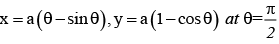Detailed Solution for Competition Level Test: Application of Derivative- 2 - Question 19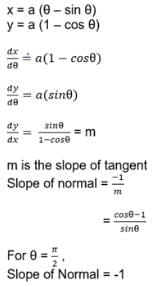Competition Level Test: Application of Derivative- 2 - Question 20

The line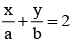is a tangent to the curve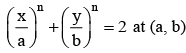then n ∈

Detailed Solution for Competition Level Test: Application of Derivative- 2 - Question 20

Calculate slope

Competition Level Test: Application of Derivative- 2 - Question 21

The points on the curve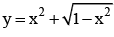at which the tangent is perpendicular to x-axis are

Detailed Solution for Competition Level Test: Application of Derivative- 2 - Question 21

dy/dx is not defined.

Competition Level Test: Application of Derivative- 2 - Question 22

The point on the curve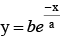at which the tangent drawn is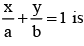Competition Level Test: Application of Derivative- 2 - Question 23

The sum of the squares of the intercepts on the axes of the tangent at any point on the curve x 2/3 + y2/3= a2/3 is

Detailed Solution for Competition Level Test: Application of Derivative- 2 - Question 23

Equation of the tangent at p (θ) to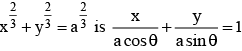Competition Level Test: Application of Derivative- 2 - Question 24

If the straight line x cos α + y sinα = p touches the curve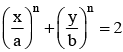at the point (a, b) on it, then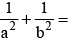Detailed Solution for Competition Level Test: Application of Derivative- 2 - Question 24

Find dy/dx and the equation of the tangent

Competition Level Test: Application of Derivative- 2 - Question 25

If the curves x = y² and xy = k cut each other orthogonally then k² =

Detailed Solution for Competition Level Test: Application of Derivative- 2 - Question 25

m1.m2 =-1

Competition Level Test: Application of Derivative- 2 - Question 26

The angle between the curves y = x³ and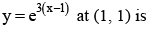Detailed Solution for Competition Level Test: Application of Derivative- 2 - Question 26

Find dy/dx to the two curves at (1, 1) they are m1 and m2. Then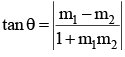Competition Level Test: Application of Derivative- 2 - Question 27

If the curves ay + x² = 7 and x³ = y cut orthogonally at (1, 1) then a =

Detailed Solution for Competition Level Test: Application of Derivative- 2 - Question 27

Slope of the first curve at (1, 1) is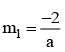Slope of the second curve at (1, 1) is m2 = 3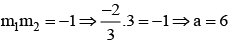Competition Level Test: Application of Derivative- 2 - Question 28

A particle moves along a line is given by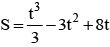then the distance travelled by the particle before it first comes to rest is

Detailed Solution for Competition Level Test: Application of Derivative- 2 - Question 28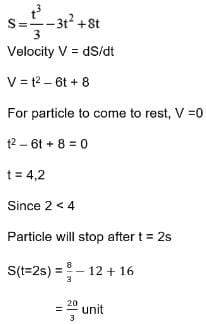Competition Level Test: Application of Derivative- 2 - Question 29

A particle is moving along a line such that s = 3t3 - 8t + 1. Find the time ‘t’ when the distance ‘S’ travelled by the particle increases.

Detailed Solution for Competition Level Test: Application of Derivative- 2 - Question 29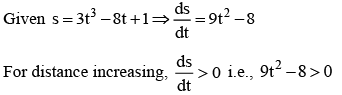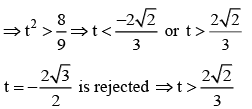Competition Level Test: Application of Derivative- 2 - Question 30

A particle moves along a line by S = t3 - 9t2 + 24t the time when its velocity decreases.

Detailed Solution for Competition Level Test: Application of Derivative- 2 - Question 30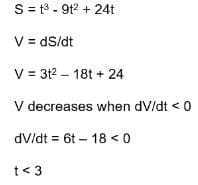## Mathematics For JEE

130 videos|359 docs|306 tests
Information about Competition Level Test: Application of Derivative- 2 Page
In this test you can find the Exam questions for Competition Level Test: Application of Derivative- 2 solved & explained in the simplest way possible. Besides giving Questions and answers for Competition Level Test: Application of Derivative- 2, EduRev gives you an ample number of Online tests for practice

## Mathematics For JEE

130 videos|359 docs|306 tests(Scan QR code)